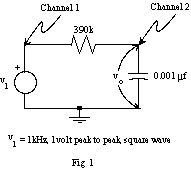EE 212 Lab
Lab 3: Square Waves and RC Time Constants

The purpose of this lab is to measure the step and square-wave responses of an RC circuit to gain a better understanding of step responses and time constants.

Laboratory Procedure:

1. Observe waveforms using the oscilloscope and note effects of AC vs. DC coupling.

• Set the frequency of your function generator to approximately 10 Hz and the waveform to a 5V peak-to-peak square-wave and then a 5V peak-to-peak triangle-wave. Set up the oscilloscope to view these waveforms.
• Note and sketch differences when the scope input is AC or DC coupled. Explain why the signal is degraded on AC coupling? Use DC coupling for the rest of this lab.

2. Measure rise time of a function generator square-wave.

• Set the frequency of a 5V peak-to-peak square-wave to 1MHz on the function generator and measure the rise-time of the square-wave (i.e., the time it takes to go from 10% to 90% of its final value while rising) using the oscilloscope by expanding the time base.

3. Calibration and probe settings for the oscilloscope.

• Display the 1kHz square-wave from the probe comp output of your scope. Adjust the trigger to obtain a stable waveform if necessary.
• Measure the amplitude of the waveform with the 10X probe in both the 1X and 10X positions.
• Compensate the probe in the 10X position to correctly view the square wave. Draw the wave shape when the probe is under-compensated and over-compensated.
• Why does one use a 10X probe? (There are at least 3 possible reasons.)

4. View and investigate RC circuit step responses.

• Construct the RC circuit shown in figure 1 on your breadboard using your function generator to generate the 1kHz, 1V peak-to-peak square-wave input v1. Display both the input v1 and output vo on the scope using Channels 1 and 2. Trigger on the rising edge of the input.• Sketch the output waveforms of a single period for signal input periods of: T = 10t,  t, and 0.1t. Label your axes. Explain the form of these plots and compare to your theoretical waveforms done in the prelab.  For which pulse length does the output most resemble the input.
• For the input period T = 10t, the initial part of the waveform is equivalent to the step response of the circuit.  Use the relationship obtained in the prelab to determine the time constant of this circuit by measuring the 10% to 90% rise time. Compare with the theoretical time constant.
• Repeat the previous two parts with the resistor and capacitor interchanged. Note this circuit setup can be thought of as similar to the AC coupling studied in part 1. Sketch the circuit, sketch the input and output waveforms as the input period is varied, and answer the following questions:
• For which pulse length does the output most resemble the input?
• Why does the range of the output waveform exceed the voltage range of the source?
• Why isn't the output amplitude constant?